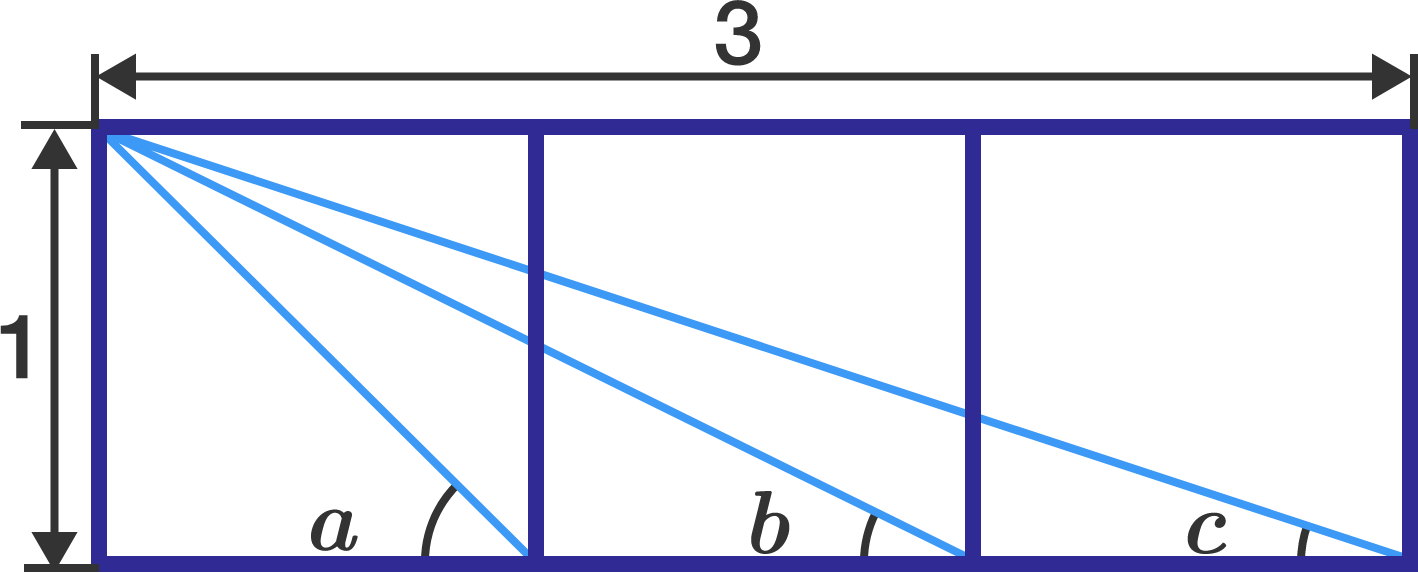# 3 Squares Puzzle

Geometry Level 2In the figure above, 3 unit squares are placed side by side. Find the sum of the angle measurements $a,$ $b,$ and $c,$ in degrees.

×